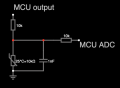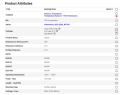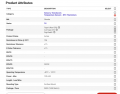# Sensor Thermistor design

#### lucasromeiro

Joined Dec 17, 2022
17
Hello guys.

I'm using an efr32bg22 Bluetooth module and would like to read a thermistor with it!

I've never used a thermistor.

Reading a little about the subject, it seems a little complicated to me.
There are some formulas for obtaining a conversion but apparently the best way is to use a conversion table.

Unfortunately I don't know how to do any of them.

I would like to use the table or a method that helps to have greater precision.

I bought 2 thermistor models and I have them here with me:
NTCSP103JF103FT1S
TX06F103F3435ER

I had a temperature sensor that used a thermistor to measure the temperature inside a refrigerator. I want to do the same thing.

I disassembled the sensor and the circuit I found was as follows:I imagine that I can replicate this circuit, but the part of converting the ADC signal to the temperature accurately I still don't know how to do it.

Can someone help me?

Important: I intend to use the range from 30 to -40 degrees celcius.
Most of the time it will operate in the range of 10 to -20 degrees celcius (inside a fridge or freezer).

Can anyone help me with this?

#### Attachments

• 2.1 MB Views: 2
• 281.9 KB Views: 2
• 523.3 KB Views: 1
Last edited by a moderator:

#### MrChips

Joined Oct 2, 2009
28,129
Reading a little about the subject, it seems a little complicated to me.
There are some formulas for obtaining a conversion but apparently the best way is to use a conversion table.
Welcome to AAC!

There is no best way to read a thermistor, just different ways to achieve the same objectives.

You stated a temperature range of -40°C to +30°C.
Next, you need to specify the desired resolution and then accuracy.

What you need to acquire is the conversion of temperature to digital counts. After you have done this you will then be able to calculate the inverse, i.e. the temperature given the digital counts.

One way to do this is by calibration. You apply a know temperature and record the digital counts. You do this for about ten pairs of data points spanning the entire desired temperature range.

Another way of doing this is by simulation. You look up the data sheet of the thermistor and find the resistance vs temperature curve. You apply this to the sensor circuit and ADC and calculate the ADC counts vs temperature.

Once you have acquired this set of data points, you perform a polynomial curve fit to the data points. Now you have an equation to convert from digital counts to temperature. You can either use the equation or you can create a lookup table. Another method is to select known points from the table and perform linear extrapolation between two points.

If I were doing this, I would not use an on-board ADC. I would create my own ADC by converting from resistance to frequency. MCUs are very good at counting with a high degree of accuracy.

#### lucasromeiro

Joined Dec 17, 2022
17
hi lucas,
Welcome to AAC.
Is this the datasheet for your version of the bgm.?
Do you have any programming experience.? it has onboard ADC,

E
View attachment 283272
Hi ericgibbs,
Yes, This is the SoC I use.
I recently read that there is a way to read the ADC in 16, 18 and 20 bit resolution.

#### ericgibbs

Joined Jan 29, 2010
17,151
•lucasromeiro

#### lucasromeiro

Joined Dec 17, 2022
17
Welcome to AAC!

There is no best way to read a thermistor, just different ways to achieve the same objectives.

You stated a temperature range of -40°C to +30°C.
Next, you need to specify the desired resolution and then accuracy.

What you need to acquire is the conversion of temperature to digital counts. After you have done this you will then be able to calculate the inverse, i.e. the temperature given the digital counts.

One way to do this is by calibration. You apply a know temperature and record the digital counts. You do this for about ten pairs of data points spanning the entire desired temperature range.

Another way of doing this is by simulation. You look up the data sheet of the thermistor and find the resistance vs temperature curve. You apply this to the sensor circuit and ADC and calculate the ADC counts vs temperature.

Once you have acquired this set of data points, you perform a polynomial curve fit to the data points. Now you have an equation to convert from digital counts to temperature. You can either use the equation or you can create a lookup table. Another method is to select known points from the table and perform linear extrapolation between two points.

If I were doing this, I would not use an on-board ADC. I would create my own ADC by converting from resistance to frequency. MCUs are very good at counting with a high degree of accuracy.
Hello MrChips,

I understood what you told me.

I would like a resolution of at least 0.1 degree celcius.

My SoC adc seems to have 12, 16, 18 and 20 bit options. Apparently it is more common to use the 16 bit. Perhaps we can use it as a reference.

As for the calculations, I know that there is an approximation that uses the Steinhart-Hart equation.

I didn't try to do a regression using datasheet points because in the places I researched people use this equation and not a polynomial regression. I don't know the reason for that...

I'm learning, I've never done anything with Thermistor.

But I'm still a little confused on the "best" way to do this.
For my use, the best way is the one that I can replicate for all my thermistors of the same model, without having to calibrate one by one and that gives me a resolution of at least 0.1 degrees calcius.

I found this material that talks a little about it and helps to calculate, but since I'm still a beginner, it left me confused.

there is an interesting table:
https://sparks.gogo.co.nz/ntc_thermistor.html

I have the thermistor datasheet:

https://www.ohmite.com/assets/docs/res_tx.pdf?r=false

https://product.tdk.com/system/file...og/tpd_automotive_ntc-thermistor_ntcsp_en.pdf#### lucasromeiro

Joined Dec 17, 2022
17

#### MrChips

Joined Oct 2, 2009
28,129
Do what eric did.
Get the datasheet of the thermistor.
Plug it into the circuit configuration and do the simulation. Get 10 pairs of data points and we will take it from there.

•lucasromeiro

#### ericgibbs

Joined Jan 29, 2010
17,151
hi lucas,
Look over this C-like Code for the Arduino, it works for me OK in the ESP32.
It uses the Steinhart-Hart equation for the thermistor.

You may have to edit to suit your C type also the NTC coefficients.

E

C-like:
/* thermistor temperature profile 17/10/2021 ESP57

Vadc = 3.3 * (Rt / (Rt + 22000))
*/
#define ADCin1    35 // ESP32 pin

float R0 = 10000;  // Therm resistance at 25C
float Bval= 3350;  // Therm Beta value
float Rth = 0;
float Rser = 22000; // Series resistor with Therm
float Vss =3.3; // ESP32 ref Volts

float TambAvg =0;

float Tref = 298.15; // 25C
float Tamb = 298.15; // 25C
int i =0;
int avg=0;

void setup()
{
Serial.begin(115200);
Serial.println("NTC1");
}

void loop() {

for (i = 0; i<= 99; i++){

// Calc Therm resistance that will give ADCvolt

//Transposed Equation for Tamb, Therm Temperature
Tamb =(Tref*Bval)/((Tref * log(Rth/R0)+Bval));
/*
Serial.print("  Rth=");
Serial.print(Rth);
Serial.print("  Cdeg=");
Serial.println((Tamb-273.15),3);
*/
TambAvg=TambAvg + Tamb;
avg=avg+1;
}

if (avg >= 99){
Serial.print ("   TambAvg=");
Serial.println((TambAvg/avg)-273.15);
TambAvg=0;
avg=0;
delay(100);}
}

#### lucasromeiro

Joined Dec 17, 2022
17
Do what eric did.
Get the datasheet of the thermistor.
Plug it into the circuit configuration and do the simulation. Get 10 pairs of data points and we will take it from there.
Hey,
Thanks!
I'll still have to learn how the software works.

#### lucasromeiro

Joined Dec 17, 2022
17
hi lucas,
Look over this C-like Code for the Arduino, it works for me OK in the ESP32.
It uses the Steinhart-Hart equation for the thermistor.

You may have to edit to suit your C type also the NTC coefficients.

E

C-like:
/* thermistor temperature profile 17/10/2021 ESP57

Vadc = 3.3 * (Rt / (Rt + 22000))
*/
#define ADCin1    35 // ESP32 pin

float R0 = 10000;  // Therm resistance at 25C
float Bval= 3350;  // Therm Beta value
float Rth = 0;
float Rser = 22000; // Series resistor with Therm
float Vss =3.3; // ESP32 ref Volts

float TambAvg =0;

float Tref = 298.15; // 25C
float Tamb = 298.15; // 25C
int i =0;
int avg=0;

void setup()
{
Serial.begin(115200);
Serial.println("NTC1");
}

void loop() {

for (i = 0; i<= 99; i++){

// Calc Therm resistance that will give ADCvolt

//Transposed Equation for Tamb, Therm Temperature
Tamb =(Tref*Bval)/((Tref * log(Rth/R0)+Bval));
/*
Serial.print("  Rth=");
Serial.print(Rth);
Serial.print("  Cdeg=");
Serial.println((Tamb-273.15),3);
*/
TambAvg=TambAvg + Tamb;
avg=avg+1;
}

if (avg >= 99){
Serial.print ("   TambAvg=");
Serial.println((TambAvg/avg)-273.15);
TambAvg=0;
avg=0;
delay(100);}
}

Hey ericgibbs,
Thanks!

Would you mind explaining to me a little how you did it?
I would like to learn how you modeled in the software and generated this code.

I think it's important to learn to use in future projects.

I'm going to build a circuit and test this software!
You helped me a lot!

#### MrChips

Joined Oct 2, 2009
28,129
I would like a resolution of at least 0.1 degree celcius.
Resolution and accuracy are two different things.

±0.1°C @ 40°C is 1 part in 400 or ±0.25%.

You are not going to get repeatable results at that accuracy with 1% components.

•lucasromeiro

#### lucasromeiro

Joined Dec 17, 2022
17
Resolution and accuracy are two different things.

±0.1°C @ 40°C is 1 part in 400 or ±0.25%.

You are not going to get repeatable results at that accuracy with 1% components.
I understand.
But, i can calibrate this, ok?

The resolution will depend on my ADC.
Maybe 16 bit is enough for 0.1C.
But as for accuracy, the 1% component doesn't help. But maybe I can fix this error.

#### MrChips

Joined Oct 2, 2009
28,129

8 bits = 1/256 = 0.4%
10 bits = 1/1024 = 0.1%
12 bits = 1/4096 = 0.024%
16 bits = 1/65536 = 0.0015%

Thus 12 bits is plenty.

If you want interchangeability without having to calibrate you need to go for 0.1% components.

#### ericgibbs

Joined Jan 29, 2010
17,151
Hi Lucas,
Look over this PDF.
E

#### Attachments

• 60.4 KB Views: 5
•lucasromeiro

#### lucasromeiro

Joined Dec 17, 2022
17

8 bits = 1/256 = 0.4%
10 bits = 1/1024 = 0.1%
12 bits = 1/4096 = 0.024%
16 bits = 1/65536 = 0.0015%

Thus 12 bits is plenty.

If you want interchangeability without having to calibrate you need to go for 0.1% components.
The 0.1% component problem is the price.
They are much more expensive. dozens of times more expensive.
I didn't find anything I could use

#### lucasromeiro

Joined Dec 17, 2022
17

#### Ian0

Joined Aug 7, 2020
6,993
First choose your pullup resistor - you chose 10k, to go with a thermistor that reads 10k at 25°C. That's perfect providing that you are most interested in temperatures around 25°C. If you want to measure a refrigerator, choose a pullup resistor that is the same as the thermistor's resistance at 4°C.
You don't need to connect the 10k resistor to an output port unless you are desperate to save power, or you want to avoid self-heating of the thermistor by the current through it. It can connect straight to the positive supply. The 10k resistor on the processor input is also unnecessary, unless your thermistor is on really long leads. If so, a capacitor across the input is not a bad idea.
Its a fair bet that your processor will have plenty of spare memory. If so, use Excel to create a spreadsheet to work out the temperature for all possible input voltages. Then turn that into a lookup table.
The relationship between voltage and temperature is so non-linear that trying to calculate it mathematically in the processor is a waste of time.